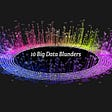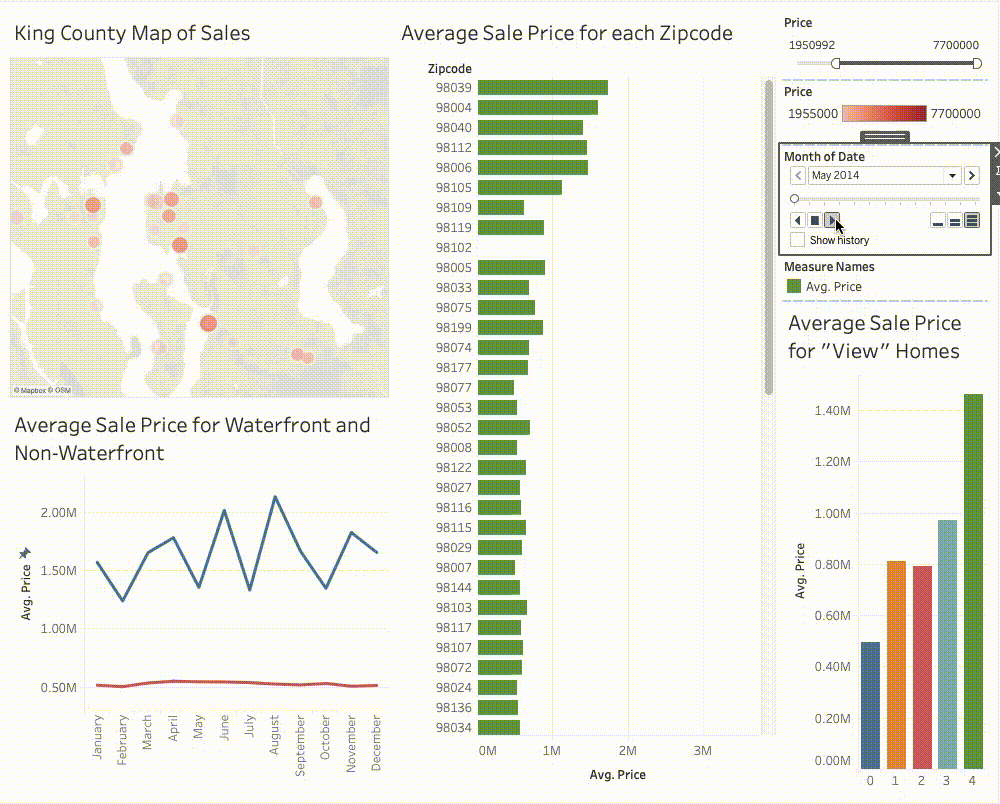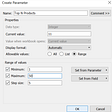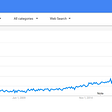# Descriptive Statistical Analysis Using Tableau

Authors: Nishu Singh & Nishanth Mannem

What is Statistical Analysis?

Statistics (or statistical Analysis) is a branch of applied mathematics that involves collecting, analyzing numerical and quantitative data to interpret patterns and trends and predict what might happen next to make better scientific opinions and conclusions. Statistical Analysis helps in collecting research interpretations, applied mathematical models, or survey studies. It can also be helpful for business intelligence organizations that have to deal with large amounts of data.

Statistical Analysis is an essential component of any business intelligence function. The demand for statistics-based functionality is increasing because it aids in examining, analyzing, and generating insights from data.

Descriptive statistics is a statistical domain that uses statistical measures of central tendency and dispersion to summarize data.

Importance of Statistical Analysis:

There are numerous approaches a business organization can use statistical evaluation to its advantage.

• Summarizing and imparting the data in a graph or chart to provide key insights.
• Calculating if the data is clustered or spread out, as well as similarities.
• Making forecasts about the future based solely on past behavior.
• Testing and sampling hypothesis from an experiment.

What is Tableau?

Tableau is a business intelligence and data visualization tool used to report and analyze large volumes of data. Tableau allows customers to create unique charts, graphs, maps, dashboards for visualizing and reading data to assist in making business decisions.

Features of Tableau:

• Tableau helps effective data discovery and exploration.
• It can connect with numerous data sources that other BI tools do not support. Tableau permits customers to create reports with the aid of joining and blending distinct datasets.
• Tableau Server supports a centralized region to control all published data sources within an organization.

Descriptive Statistics using Tableau:

In the right hands, data can be highly powerful and a vital factor in making decisions. To examine data and make educated decisions, we can use statistical metrics. Tableau allows us to compute various statistical measurements like Mean, Median, Mode, standard deviation, etc.

Using the Climate Dataset, we will analyze the terms in descriptive statistics by deriving some meaningful insights. We have used weather data of the top 8 Indian cities as per population. Datasets contain hourly weather data from January 2009 to January 2020. Details of each town are over ten years old. This data is used to make observations that will help to understand climate trends across different cities.

Mean: The ratio of the sum of all observations in the data to the total number of observations is called the mean. Therefore, the mean is a value around which all data is distributed.

We can show the average trend line in Tableau by dragging the average line from the analytics pane. We can also use the average aggregation function to calculate the mean.

When we analyze the rainfall measured by precipitation value across different metro cities, we can see that Mumbai, on average, receives more rainfall than other cities, and New Delhi receives the lowest rainfall.

Fig. 1: Bar Chart showing cities receiving rainfall above mean rainfall

Median: The Median is the point at which all data is divided into two halves. Half of the data is below the Median, the other half above it.

We can show the median trend line in Tableau by dragging the Median with quartiles from the analytics pane; we can also calculate the Median by using the median aggregation function.

Suppose we plot the average rainfall of different metropolitan cities along with the median rainfall. In that case, we can see that only Mumbai and Pune have received rainfall greater than the median values of the other three metro cities.

Fig. 2: Bar Chart showing cities receiving rainfall above-median rainfall

Mode: Mode is the value that occurs most often in the total data set, or in other words, the mode is the value with the highest frequency. The aggregation functions available in Tableau make it easier to calculate the mode.

We had used a count aggregation function to see the city-wise sunrise time when sunrise occurred with a maximum frequency in five metropolitan cities for the last four years.

The following visualization shows the maximum number of days sunrise has happened at 6:09 am in Bengaluru. For Hyderabad maximum number of days, sunrise took place at 6:59 am, similarly for other cities. We can also observe that sunrise happens quite early in New Delhi, i.e., at 5:23 am, whereas sunrise for Pune has been quite late at 7:10 am for maximum days in the last four years.

Fig. 3: Lollipop Chart showing city-wise sunrise timing at which sunrise has happened with the maximum frequency

Standard Deviation: The standard deviation is a metric for quantifying the amount of variance in a set of data values from the mean. A variable with a low standard deviation has data points close to the mean and vice versa. We can calculate the standard deviation by either using the standard deviation aggregation function or by using the standard deviation from the distribution band in the analytics pane.

We have used standard deviation to see a variation in the wind chill temperature and find the points where the wind temperature varied significantly from the average wind temperature. The months where the wind temperature was greater than one standard deviation are shown in orange color, and those within one standard deviation are shown in blue.

Fig. 4: Line chart showing year-wise wind chill variation for metropolitan cities in different years

Quartile: A quartile is an applied mathematical term that describes the distribution of sightings in four periods defined based on data values and comparisons with all observations. The dataset is split into four equal quartiles. Q1 is the first quartile of the dataset, and Q2, Q3 represents the second and third quartiles of the data set.

25% of the data points lie below Q1, and 75% lie above it.

50% of the data points lie below Q2, and 50% lie above it. Q2 is nothing but Median.

75% of the data points lie below Q3, and 25% lie above it.

Box plots help to study:

• Degree of variation

• Outliers

• Propagation for the center of data

• Comparison of data sets

• Skewness

In Tableau, we can calculate quartiles using box plots with required fields or using the quartiles from the analytics pane. We have plotted the number of sun hours for metropolitan cities in the monsoon season. We can see the maximum hours for New Delhi, which was between 12 to 14 hours for maximum days. For Pune, the sun hours have been minimum as 50% of the time the sun hours have been between 7 to 9 hours. We can also see very few days have sun hours as low as 6 for Delhi. Such points are identified as outliers.

Fig. 5: Box Plot showing Sunhours for different metropolitan cities in monsoon season

Skewness: The degree of skewness in a probability distribution is defined by skewness. It can be positive or negative.

Positive Skewness — This is the case when the curve’s tail on the right side is larger than the tail on the left. In this case, the mean of the distribution is greater than the mode.

Negative Skewness — This is when the tail on the left side of the curve is larger than the tail on the right. In this distribution, the mean is smaller than the mode.

A normal distribution (bell curve) indicates zero skewness.

From the box plot in above viz (Fig. 5) of sun hours for different cities, we can see that the Median for Pune is close to the first quartile for Pune; hence it is positively skewed, whereas for Bengaluru, the Median is close to the upper quartile hence it is negatively skewed.

Analyzing minimum, maximum, and outliers using control charts: We can find the maximum, minimum, and outliers in Tableau. We can use the distribution band from the analysis pane or use the aggregation functions for the same.

We have plotted the wind chill temperature for different months of the year. The plot shows maximum and minimum values. We can also display the upper and lower bounds using the distribution band from the analysis pane. This will help us find outliers (Points above the upper bound show outliers). We can see that the April, May, and June months have been above the upper bound value for wind temperature.

Fig. 6: Control chart to analyze the wind chill temperature across different months of the year

Now that we have explored the statistical terms, we get the following dashboard by clubbing together all the visualizations.

Fig. 7: Descriptive Statistical Analysis using Tableau

Conclusion:

Tableau is a convenient tool to perform statistical data analysis. Tableau has detailed functionalities for implementing statistical Analysis on a given dataset. The built-in statistical functions help better understand data by analyzing the trends, summarizing data, and exploring the datasets seamlessly.
Dataset: https://www.kaggle.com/hiteshsoneji/historical-weather-data-for-indian-cities

## More from Apisero

Love podcasts or audiobooks? Learn on the go with our new app.

## 10 big data blunders businesses should avoid (Top 10 Mistakes)## Data Science for the Experienced. How to Find Your Way into the World of Data Science?## What It Really Means to Gather Online Qual at Scale## Classifying Political Social Media Posts with Natural Language Processing## Getting The Most Out Of Your Tracking App, Part II: GPS Analysis## Deep Brew: Transforming Starbucks into an AI & data-driven company## Learning about Spiking Neural Networks for time-based data at the Grace Hopper Celebration 2020## When it Comes to Data Literacy, I Don’t Like Your Odds## Animating Housing Data with Tableau## Tableau Parameters## Importance of BI and Why Tableau is the best BI Tool to visualise data?## Power BI: How to Implement Advanced Analytics with Data Integration?